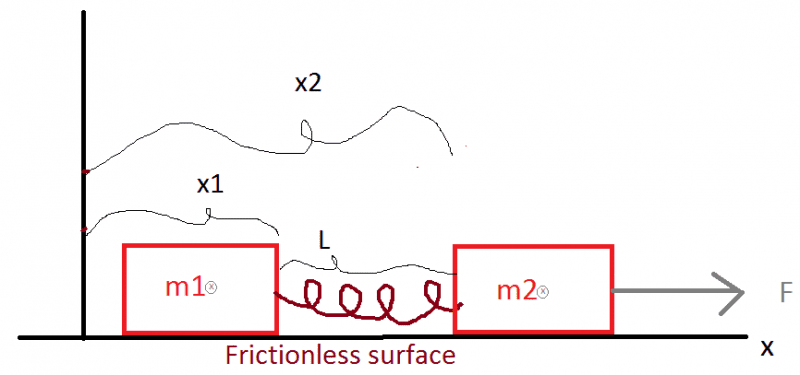# Solve Box-Spring Problem: Setup Differential Equation

• havsula
I'm not sure.In summary, the goal is to find the solutions to the system of equations:x_1(t) = ?x_2(t) = ?.

## Homework Statement

Have to boxes on a frictionless surface, connected with a massless spring with unstrecht length L and spring constant k. The boxes have mass m1 and m2 and ca be regarded as point masses.
The two variables x1 and x2 is the position of the boxes at the location where the spring are attached.

I try to set up the differential equation for this problem, but cannot manage to to that.

I think that when the force is put on, box 2 will accelerate faster than box 1 in the start, until the spring have streched enough to balacne the force F?F_spring = kdx

## The Attempt at a Solution

x1'' = k(x2-x1-L)
x2'' = -k(x2-x1-L) +F

Hi Havsula, and welcome to PF :)

I think you're doing just fine in setting up the differential equations, except you want a force on the left too: m1x1'' etc.

How does the exercise continue ? Or is all you have to do this setting up the differential equations ?

Re accelerations: you can now write expressions for a1 and a2 and that means you can also write the condition for a1 < a2

Hello
Yes the equation should be like this:

m1*x1'' = k(x2-x1-L)
m2*x2'' = -k(x2-x1-L) +F

Or in fancy latex

$m_1\frac{d^2x_1}{dt^2}-kx_2+kx_1-kL=0$

$m_2\frac{d^2x_2}{dt^2}+kx_2-kx_1+kL-F=0$

The goal is to find the solutions:

$x_1(t) = ?$
$x_2(t) = ?$

Well, now it is mathematics, not physics any more :)
How do you normally deal with such systems of differential equations ?

Anything known of F ? For example that it's constant ?
Any inital conditions given ? If not, you can assign symbols to them.

Hello
Yes it is more math now. I have only dealt with vibration problems until now, and there I know the solution. But the solution of this problems is not some functions of sinus.

F is constant.
Inital conditions is that the system is at rest and that x1 = 0 and x_2 =L (i.e the spring is unstretched).

Doesn't it look tempting to add the two equations ?

Probably. Do be honest, my math is so rusty that I need to spent some time to search for a solution method. Do you have any tips on where to start?

Yes, add the two equations :)

I have added the two equation, and to my supprise the spring data dissapeard

$m_1\frac{d^2x_1}{dt^2}+m_2\frac{d^2x_2}{dt^2}=F$

I really do not understand this problem any more.
I will believe that that the acceleration of box 1 will start from zero and gradually increase to a = F/(m1+m2) and that the spring constant will decide how fast this will happend.
What will happened with this system if a force F is suddently put on box 2?

That is exactly what you are to find out in this exercise !
And, of course, I don't want to spoil the fun of such a discovery.

But what you have found already is that this system of two masses, connected by a spring (the spring force is an internal force in this system) behaves as a mass ##m_1+m_2## located at its center of mass under the influence of the external force: $$m_1\frac{d^2x_1}{dt^2}+m_2\frac{d^2x_2}{dt^2}=F\ \ \Rightarrow\ \ (m_1+m_2)\;{m_1\frac{d^2x_1}{dt^2}+m_2\frac{d^2x_2}{dt^2}\over m_1 + m_2} = F$$With coordinate of center of mass $$\xi \equiv {m_1x_1 + m_2 x_2 \over m_1 + m_2}\;,$$what do you get ?

PS have to go, running late.

Okay
So I actually get with this:

##(m_1+m_2) \frac{d^2}{dt^2} \left( \frac{ m_1x_1 +m_2x_2 }{m_1+m_2 } \right) = F##
Changing to center of gravity:

##(m_1+m_2) \frac{d^2\xi }{dt^2} = F##

Which have this solution
##\xi(t) = \frac{F}{2(m_1+m_2)}t^2##

But I really do not understand how to get the solution I want from this.
But now I have to go to bed. Have to think more tomorrow.

havsula said:
##\xi(t) = \frac{F}{2(m_1+m_2)}t^2##
Right. Do you see how you could have written that down immediately?

##\xi(t) = {1\over 2} \frac{F}{(m_1+m_2)}t^2## still needs a ##+ \xi(0)##, which is not zero, to satisfy ##x_1(0) = 0## and ##x_2(0) = L##.

Yes that is true. With ##x_1(0)=0## and ##x_2(0) =L ## we got:
##\xi(t) = {m_2L\over m_1+m_2} {1\over 2} + \frac{F}{(m_1+m_2)}t^2##

But now I have to find out how to get from this, to the position of ##x_1## and ##x_2## as a function of time. Have to think..

You have a solution for one linear comination of the two x. That gives one equation with two unknowns. You need one more to establish both. Most likely one that has to do with the spring ...
Either that or one of the original two equations.

havsula said:
Yes that is true. With ##x_1(0)=0## and ##x_2(0) =L ## we got:
##\xi(t) = {m_2L\over m_1+m_2} {1\over 2} + \frac{F}{(m_1+m_2)}t^2##

But now I have to find out how to get from this, to the position of ##x_1## and ##x_2## as a function of time. Have to think..
I would rewrite your original differential equation (either one) in terms of the distance from the common mass centre to one of the masses.

BvU said:
You have a solution for one linear comination of the two x. That gives one equation with two unknowns. You need one more to establish both. Most likely one that has to do with the spring ...
Either that or one of the original two equations.
Yes, I have come to that conclusion. Now I only have to figure out how these equation look like. Will be back when I have something:)